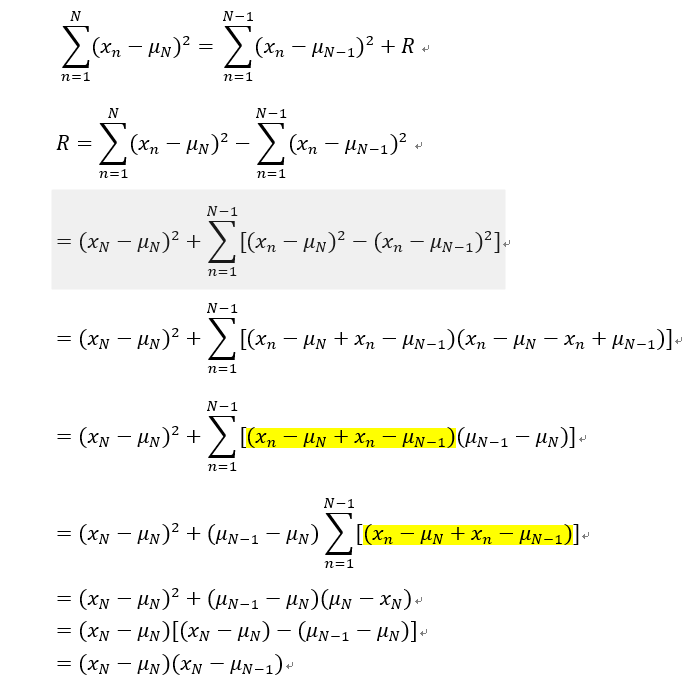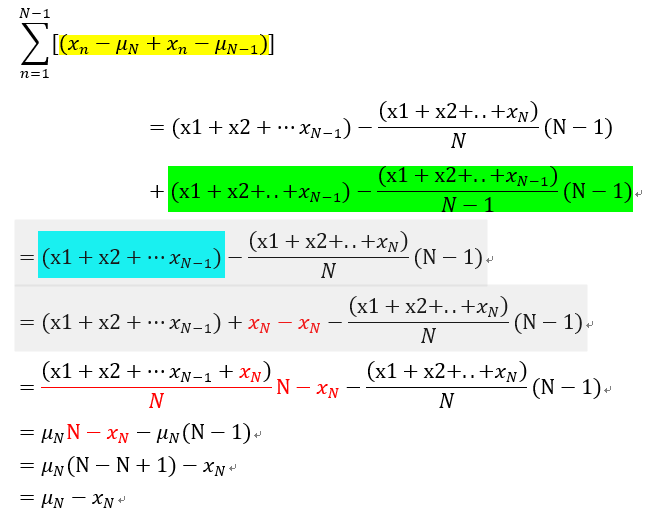#2019 iT 邦幫忙鐵人賽DAY 5
0

## Day 5-機器學習與數學天天玩-PCA-Statistical Introduction: Welford’s Method

The brief structure leading to the milestone of PCA is as below:
1. Statistical Introduction
2. Transformation of Vectors in Spaces
3. Orthogonal Projectio

Hi everyone, after a practice in the course, I found a very interesting method applied to explore the concept of variance. To understand the impact on a variance after adding a new data point into a dataset, a method called Welford’s Method would help a lot.

Below is how we get the difference between the two variances where one is with N sample and the other is with N-1 sample.A part of the above formula marked yellow is further dissected below:The process of inference lets us see the beautiful technique we can use in mathematics.
Think and move forward together!